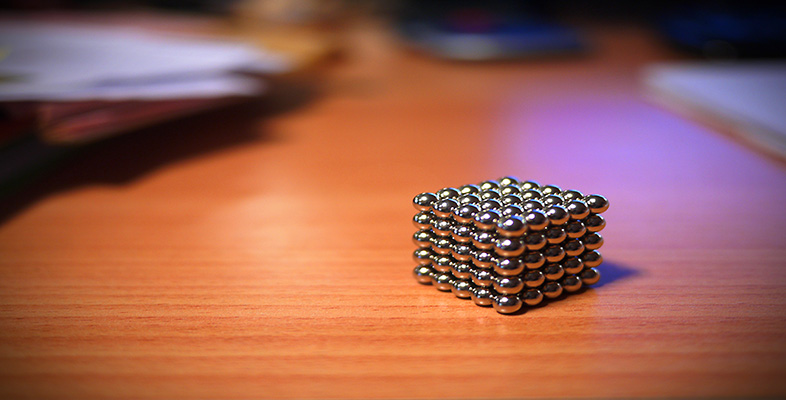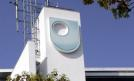Squares, roots and powers

This free course is available to start right now. Review the full course description and key learning outcomes and create an account and enrol if you want a free statement of participation.

Free course

# 1.4.1 Try some yourself

## Activity 8

If a litre is one cubic decimetre, how many litres are there in a cubic metre?

Since a litre is one cubic decimeter, the question is asking how may cubic decimetres are in a cubic metre.

1 metre = 10 decimetres

so 1 m3 = 103 cubic decimetres.

Hence there are 1000 litres in a cubic metre.

## Activity 9

Find the following without using your calculator, as an estimate for the calculator work in the next question.

• (a) (1)3

• (b) 33

• (c) 1003

• (d) 0.13

• (a) (1)3 = 1 × 1 × 1 = 1 × 1 = 1

• (b) 33 = 3 × 3 × 3 = 9 × 3 = 27

• (c) 1003 = 100 × 100 × 100 = 10 000 × 100 = 1000 000 (a million)

• (d)## Activity 10

Use your calculator to find the following.

• (a) (1.2)3

• (b) 3.33

• (c) 1013

• (d) 0.1213

• (a) Estimate: (1)3 = 1.

Calculate: (1.2)3 = 1.728.

• (b) Estimate: 33 = 27.

Calculate: 3.33 = 35.937.

• (c) Estimate: 1003 = 1000 000.

Calculate: 1013 = 1030 301.

• (d) Estimate: .13 = .001.

Calculate: 0.1213 = 0.00177 (3 s.f.)

## Activity 11

What are the following?

• (a)• (b)• (a)= 1 since 13 = 1.

• (b)= 10 since 103 = 1000.

## Activity 12

Find the volume of a one-foot cube in cubic metres (1 foot = 30.48 cm). Estimate your answer first. Round your answer to three decimal places.

To estimate an answer, choose a simple approximation, say

1 foot30 cm = 0.3 m.

Then 1 foot cubed(0.3)3 = 0.027 m3.

More accurately, 1 foot = 30.48 cm = 0.3048 m.

So 1 foot cubed = (0.3048)3 = 0.028 m3 to three d.p.

## Activity 13

Without using your calculator, find the following, as estimates for the calculator work in Question 7.

• (a) 92

• (b) 43

• (c)• (d)• (e) (3)2

• (a) 92 = 9 × 9 = 81

• (b) 43 = 4 × 4 × 4 = 16 × 4 = 64

• (c)= 8 (since 82 = 64)

• (d)• (e) (3)2 = 3 × 3 = 9

## Activity 14

Use your calculator to find:

• (a) 9.422

• (b) 3.653

• (c)• (d) 0.333

• (e) (2.713)2

Use your answers to the previous question as rough checks. Round your answers to four decimal places.

• (a) 9.422 = 88.7364

• (b) 3.65348.6271

• (c)8.3666

• (d) 0.3330.0359 (check this by calculating, the estimate from 3(d) above, as a decimal.

• (e) (2.713)27.3604 (N.B. To get this answer on a calculator, remember the brackets.)

MU120_4M4

### Take your learning furtherMaking the decision to study can be a big step, which is why you'll want a trusted University. The Open University has nearly 50 years’ experience delivering flexible learning and 170,000 students are studying with us right now. Take a look at all Open University courses.

If you are new to university level study, find out more about the types of qualifications we offer, including our entry level Access courses and Certificates.

Not ready for University study then browse over 900 free courses on OpenLearn and sign up to our newsletter to hear about new free courses as they are released.

Every year, thousands of students decide to study with The Open University. With over 120 qualifications, we’ve got the right course for you.

Request an Open University prospectus